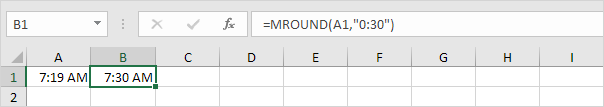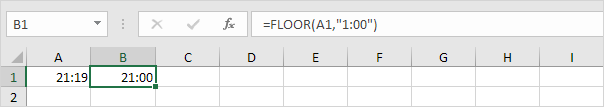# Rounding Times

Use MROUND in Excel to round a time to the nearest 15 minutes, the nearest 30 minutes, the nearest hour, etc.

1. The formula below rounds a time to the nearest 15 minutes.Note: if your formula returns a decimal number, don’t panic. Times are handled internally as numbers between 0 and 1. Simply apply a Time Format to display a time.

2. The formula below rounds a time to the nearest 30 minutes.3. The formula below rounds a time to the nearest hour.4. FLOOR always rounds down. The formula below rounds a time down to the nearest hour.5. CEILING always rounds up. The formula below rounds a time up to the nearest hour.Note: change the Time Format to display a time without AM/PM (24 Hour Time Format).

6. Use the “:” (colon) twice to enter a time with seconds in Excel. For example, 2 hours, 43 minutes and 15 seconds.7. The CEILING function below rounds this time up to the next minute.Tip: use the Format Painter to quickly copy the format of cell A1 to cell B1.